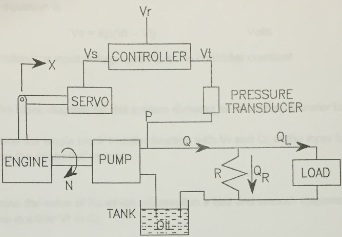### Determine the single block transfer function with vr and ql

Assignment Help Mechanical Engineering
##### Reference no: EM131008881

A proportional controller is to be used to control the pump output pressure in the system shown belowThe steady state output torque of the engine is given by the equation

Tss = 1500 X - 25 N fit-lbf

where X is the throttle position in inches and N is the engine speed in rpm. The total combined rotational moment of inertia (I) of the engine and pump combined is 150 ft¬lbf-s2. The output of the pump is related to the engine speed by the equation

Q = 0.1 N gal/min

where N is in rpm. The torque required to drive the pump is determined by the pressure output from the pump

T = 0.5 P ft-lbf

Where P is in psi. The bypass circuit has a constant resistance R of 300 psi/(gal/min).

The voltage output of the pressure transducer is given by the equation

Vt = 0.0001 P Volts

Where P is in psi. The throttle servo is a first order system with a time constant of 1 second and a steady state responsegiven by the equation below.

Xss = 0.5 Vs inches

Where Vs is the voltage input to the servo.

The controller equation is

Vs = Kp(Vr Vt) Volts

where Vr is a reference input voltage and Kp is the controller constant

1. Draw the block diagram for this system showing all necessary transfer functions

2. Determine the single block transfer function with Vr and QL in the input function and P as the controlled variable.

3. Determine the value of Kp which will result in a fast and smooth response to changes in either Vr or QL.

4. Determine the equation for the steady state value of P for given values of Vr and QL using your value of Kp.

5. The system is operating at steady atate with Vr equal to 2 volts and QL equal to 0. QL suddenly changes to 25 gal/min and remains fixed at that value. Plot X, P, and N against time during the transition to a new steady state. These plots may be determined from an exact solution of the differential equation or a numerical solution based in the system equations. A listing of the program with comments explaining the solution must be included with the solution.

#### How many atoms are there per unit cell

A 3-D cubic unit cell contains four atoms whose centers are positioned halfway up along each of the vertical cell edges and one atom each centered in the middle of the top a

#### Determine the width w of the link bc

Determine the width w of the link BC needed so that the structure with load P = 20 kips has a factor of safety equal to 2.5. Link BC is ½ in thick and has a 85 ksi ultimate st

#### Estimate the maximum power output

A dam is proposed on a remote stream that measures approximately 25-cm deep by 350-cm wide with an average velocity of 2.2 m=s. If the dam can be constructed so that the fre

#### Obtain the compressive stresses sab and sbc in the two parts

A two-story column ABC in a building is constructed with a hollow, square box section (see figure). The outside dimensions are 200 mm x 200 mm, and the wall thickness is 15

#### Pipes are horizontal bad viscous effects are negligible

Gasoline flows from a 0.25 m diameter pipe in which the pressure is 250 kPa, that contracts into a 0.125 m diameter pipe in which the pressure is 100 kPa. If the pipes are hor

#### What is the specific volume

what is the specific volume, internal energy (kj/kg) and enthalpy (kj/kg) for water at 1.8 mpa and 120 Degrees C? Locate the state on p-v diagram and document assumptions.

#### What is the convection coefficient of water flowing in tube

Saturated liquid water at 30°C and 1.8 m/s enters a long brass (k = 108 W/m-K) condenser tube with inner diameter of 1.45 cm and outer diameter of 1.6 cm. The heat transfer co

#### Maximum total deflection

A helical spring is to be constructed from a 4340 steel. The design calls for 5 coils, a coil-to-coil diameter of 12 mm, and a wire diameter  of  3  mm. Furthermore, a  maxi

### Write a Review